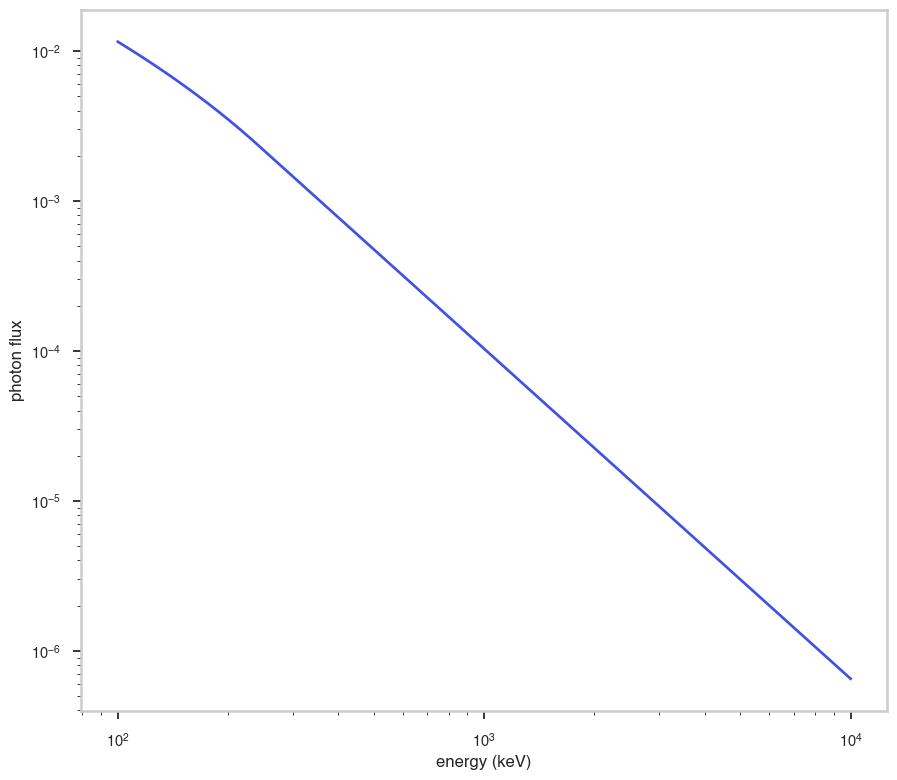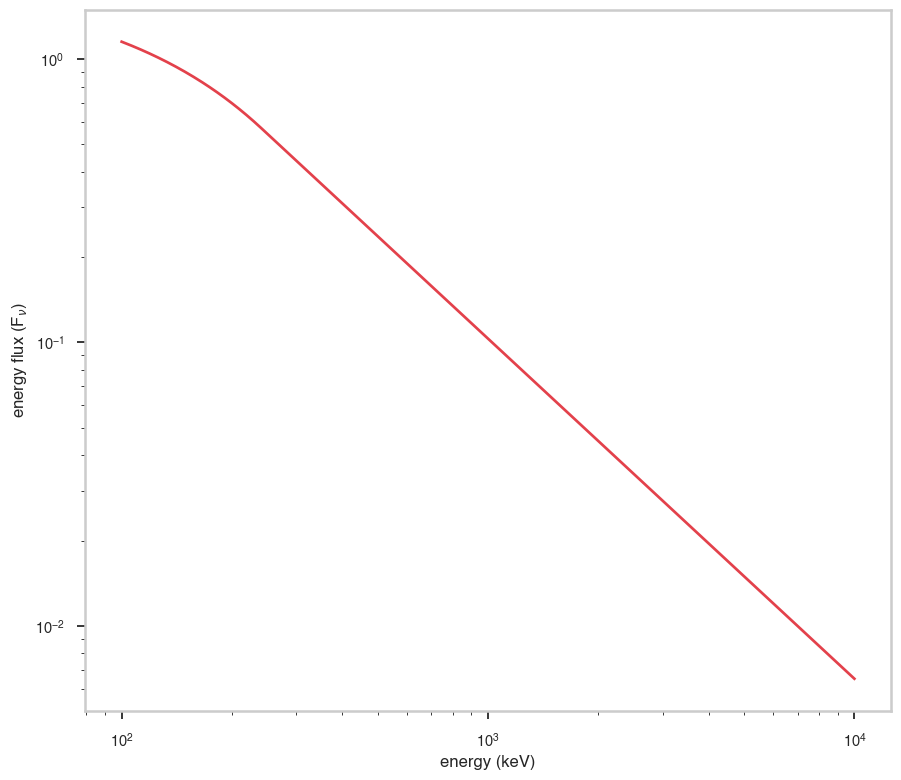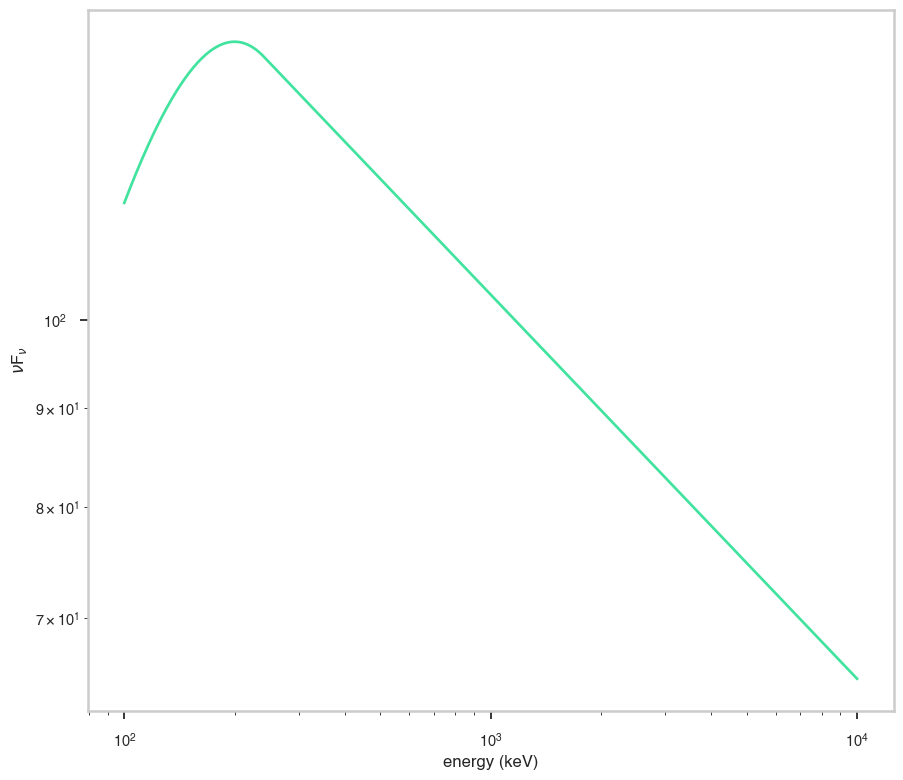# Band Calderone

:

# Parameters
func_name = "Band_Calderone"
wide_energy_range = True
x_scale = "log"
y_scale = "log"
linear_range = False


## Description

:

func.display()

• description: The Band model from Band et al. 1993, implemented however in a way which reduces the covariances between the parameters (Calderone et al., MNRAS, 448, 403C, 2015)
• formula: $\text{(Calderone et al., MNRAS, 448, 403C, 2015)}$
• parameters:
• alpha:
• value: -1.0
• desc: The index for x smaller than the x peak
• min_value: -10.0
• max_value: 10.0
• unit:
• is_normalization: False
• delta: 0.1
• free: True
• beta:
• value: -2.2
• desc: index for x greater than the x peak (only if opt=1, i.e., for the Band model)
• min_value: -7.0
• max_value: -1.0
• unit:
• is_normalization: False
• delta: 0.22000000000000003
• free: True
• xp:
• value: 200.0
• desc: position of the peak in the x*x*f(x) space (if x is energy, this is the nuFnu or SED space)
• min_value: 0.0
• max_value: None
• unit:
• is_normalization: False
• delta: 20.0
• free: True
• F:
• value: 1e-06
• desc: integral in the band defined by a and b
• min_value: None
• max_value: None
• unit:
• is_normalization: True
• delta: 1e-07
• free: True
• a:
• value: 1.0
• desc: lower limit of the band in which the integral will be computed
• min_value: 0.0
• max_value: None
• unit:
• is_normalization: False
• delta: 0.1
• free: False
• b:
• value: 10000.0
• desc: upper limit of the band in which the integral will be computed
• min_value: 0.0
• max_value: None
• unit:
• is_normalization: False
• delta: 1000.0
• free: False
• opt:
• value: 1.0
• desc: option to select the spectral model (0 corresponds to a cutoff power law, 1 to the Band model)
• min_value: 0.0
• max_value: 1.0
• unit:
• is_normalization: False
• delta: 0.1
• free: False

## Shape

The shape of the function.

If this is not a photon model but a prior or linear function then ignore the units as these docs are auto-generated

:

fig, ax = plt.subplots()

ax.plot(energy_grid, func(energy_grid), color=blue)

ax.set_xlabel("energy (keV)")
ax.set_ylabel("photon flux")
ax.set_xscale(x_scale)
ax.set_yscale(y_scale)## F$$_{\nu}$$

The F$$_{\nu}$$ shape of the photon model if this is not a photon model, please ignore this auto-generated plot

:

fig, ax = plt.subplots()

ax.plot(energy_grid, energy_grid * func(energy_grid), red)

ax.set_xlabel("energy (keV)")
ax.set_ylabel(r"energy flux (F$_{\nu}$)")
ax.set_xscale(x_scale)
ax.set_yscale(y_scale)## $$\nu$$F$$_{\nu}$$

The $$\nu$$F$$_{\nu}$$ shape of the photon model if this is not a photon model, please ignore this auto-generated plot

:

fig, ax = plt.subplots()

ax.plot(energy_grid, energy_grid**2 * func(energy_grid), color=green)

ax.set_xlabel("energy (keV)")
ax.set_ylabel(r"$\nu$F$_{\nu}$")
ax.set_xscale(x_scale)
ax.set_yscale(y_scale)